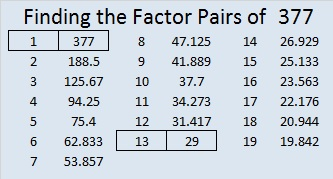# 377 and Level 1

377 isn’t even, and the sum of its digits don’t add up to a multiple of 3. I’ll give it the quick prime number test: 2^377 (mod 377) = 188, not 2, so 377 is definitely a composite number. Scroll down past the puzzle to see its factoring information.

Print the puzzles or type the factors on this excel file: 10 Factors 2015-02-02

• 377 is a composite number.
• Prime factorization: 377 = 13 x 29
• The exponents in the prime factorization are 1 and 1. Adding one to each and multiplying we get (1 + 1)(1 + 1) = 2 x 2 = 4. Therefore 377 has exactly 4 factors.
• Factors of 377: 1, 13, 29, 377
• Factor pairs: 377 = 1 x 377 or 13 x 29
• 377 has no square factors that allow its square root to be simplified. √377 ≈ 19.41613 and 29 are both Pythagorean triple hypotenuses so 377 can be written as the sum of two squares two different ways, and it is the hypotenuse of FOUR Pythagorean triples, half of which are primitives:

• 19² + 4² = 377
• 16² + 11² = 377
• 135² + 352² = 377²
• 145² + 348² = 377²
• 152² + 345² = 377²
• 260² + 273² = 377²

## One thought on “377 and Level 1”

1.Paula Beardell Krieg

It just seems inconvenient to have 13 and 29 as factors. There are times when I can relate to 377.

This site uses Akismet to reduce spam. Learn how your comment data is processed.